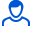Content: 40988.png (51.49 KB)

Positive responses: 0
Negative responses: 0

Sold: 1
Refunds: 0Loyalty discount! If the total amount of your purchases from the seller more than:

 \$1 the discount is 10% \$5 the discount is 30%

The physical pendulum is a heterogeneous disk mounted on an axis. The pendulum performs small oscillations around a horizontal axis with a frequency of 15 rad / s. If a small load of mass m = 50 g is attached to it at a distance l = 20 cm below the axis, the oscillation frequency becomes equal to 10 rad / s. Determine the moment of inertia of this pendulum relative to the axis of swing.
Task 40988. Detailed solution with a brief record of the conditions, formulas and laws used in the decision, the conclusion of the calculation formula and the answer.
If you have any questions about the decision, write. I will try to help. File in image format.
No feedback yet